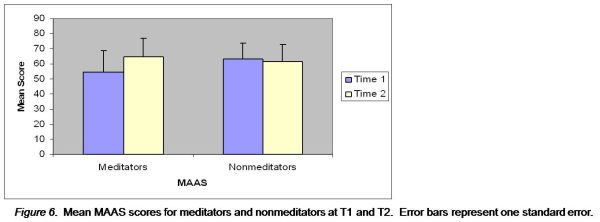# Changes on Measures of Metacognitive Processing

### Richard Chambers

To determine whether the meditation course successfully enhanced participants' metacognitive processing capacity (Hypothesis 2), participants' scores before and after the intervention on the RRS and MAAS were analysed by two-way 2 (time) by 2 (group) ANOVAs.

RRS. Figure 5 represents the mean RRS scores, for each group, at T1 and T2. The meditators demonstrated a significant decrease over the course of the intervention (t = 2.78, p = .01, d = .46), while the nonmeditators demonstrated no significant decrease (t = 1.24, p = .23, d = .13).There was a highly significant main effect of time (F[1,38] = 8.97, p = .005, â•¬Ã€2 = .19), a nonsignificant main effect of group (F<1, â•¬Ã€2 < .001), and a nonsignificant time by group interaction (F[1,38] = 2.49, p = .12, â•¬Ã€2 = .06).

MAAS. Figure 6 represents the mean MAAS scores, for each group, at T1 and T2. The meditators demonstrated a highly significant increase over the course of the intervention (t = -4.47, p < .001, d = -.76), while the nonmeditators demonstrated no significant increase (t = 1.48, p = .16, d = .15).There was a highly significant main effect of time (F[1,38] = 11.28, p = .002, â•¬Ã€2 = .23), a nonsignificant main effect of group (F[1,38] = .66, p = .42, â•¬Ã€2 = .02), and a highly significant time by group interaction effect (F[1,38] = 21.75, p < .001, â•¬Ã€2 = .36).